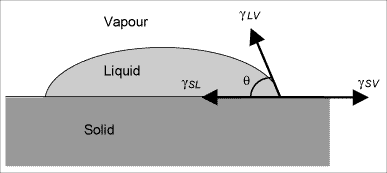Subscribe to our newsletter to receive the latest news and events from TWI:

# What is meant by wetting and how can it be used?

The wetting of surfaces by liquids, which includes adhesives, can be understood by considering a droplet of liquid resting on a surface. The wetting action can be quantified using the various tensions that act at the point where the three phases, of liquid, solid and vapour, meet, as shown below. The tensions, or surface tensions, are represented by the symbol γ, and the subscripts L, S and V stand for liquid, solid and vapour. The surface tensions can be combined with the equilibrium contact angle, γ LV cos θDiagram showing the relationship for the three surface tensions (surface free energies) for a droplet of liquid resting on a solid substrate at the three-phase point

The surface tension, or surface free energy, γ, of a material can thus be obtained by measuring the contact angles of liquids on the surface of the material, and from knowing the surface free energies of the liquids, which can be found in tables of chemical and material properties.

The Young equation (γ SL+γ LV COS Ø=γ SV) makes several key assumptions, such as a perfectly flat surface and an ideal liquid, and it should be remembered that real liquids and surfaces might differ significantly from this ideal. To be strictly accurate, the surface free energy, γ SV , measured in this way is actually the value resulting from absorption of vapour from the liquid onto the solid material, and is a lower value than the true surface energy γ S that would be measured in a vacuum. However, other potential sources of error, such as surface roughness and changing contact angle, means that in most practical cases the γ SV value is the one used and quoted.